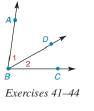Chapter 1.2, Problem 43E### Elementary Geometry for College St...

6th Edition
Daniel C. Alexander + 1 other
ISBN: 9781285195698

#### Solutions

Chapter
Section### Elementary Geometry for College St...

6th Edition
Daniel C. Alexander + 1 other
ISBN: 9781285195698
Textbook Problem
1 views

# In Exercises 41 to 44, m ∠ 1 + m ∠ 2 = m ∠ A B C .Find x if m ∠ 1 = x , m ∠ 2 = 2 x + 3 , and m ∠ A B C = 72 ° .To determine

To find:

x for m2=x, m1=2x+3, and mABC=72°.

Explanation

Calculation:

Given,

m2=x,

m1=2x+3, and

mABC=72°.

m1+m2=mABC

Substitute;m2=x, m1=2x+3, and mABC=72° in the above relation,

2x+3+x=72

### Still sussing out bartleby?

Check out a sample textbook solution.

See a sample solution

#### The Solution to Your Study Problems

Bartleby provides explanations to thousands of textbook problems written by our experts, many with advanced degrees!

Get Started

#### Evaluate the definite integral. 01(3t1)50dt

Single Variable Calculus: Early Transcendentals

#### Expand each expression in Exercises 122. (y1y)(y+1y)

Finite Mathematics and Applied Calculus (MindTap Course List)

#### Evaluate the limit, if it exists. limt3t292t2+7t+3

Single Variable Calculus: Early Transcendentals, Volume I

#### Sometimes, Always, or Never: If c is a critical number, then f′(c) = 0.

Study Guide for Stewart's Single Variable Calculus: Early Transcendentals, 8th